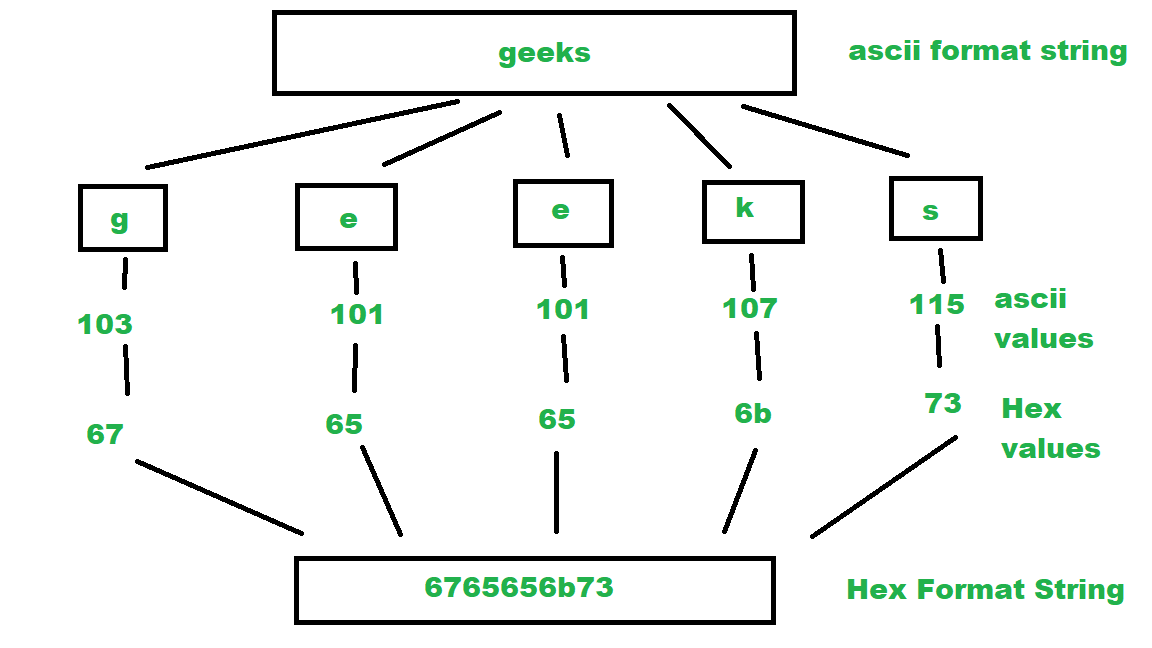# Convert a string to hexadecimal ASCII values

Given a string as input, write a program to convert the characters of given string into hexadecimal equivalent of ASCII values.
Examples :

```Input : Geek
Output : 4765656b

Input :  IronMan part 3
Output : 49726f6e4d616e20706172742033
```

## Recommended: Please try your approach on {IDE} first, before moving on to the solution.

ASCII stands for American Standard Code for Information Interchange. ASCII is a standard that assigns letters, numbers, and other characters within the 256 slots available in the 8-bit code. E.g the lower case “h” character (Char) has a decimal value of 104, which is “01101000” in binary and “68” in hexadecimal.ASCII TABLE

The “Hexadecimal” or simply “Hex” numbering system uses the Base of 16 system. Hexadecimal number uses 16 symbols {0, 1, 2, 4, 5, 6, 7, 8, 9, A, B, C, D, E, F} to represent all numbers. Here, (A, B, C, D, E, F) represents (10, 11, 12, 13, 14, 15).

Algorithm :

1. Initialize final Hex string as empty.
2. Consider every character from input, cast it into integer. This integer value is ascii value of that character.
3. Change this integer value into hexadecimal value and add this hexadecimal value to final Hex string.Basic implementation of the above idea:

 `// Java program to convert ASCII ` `// string to Hexadecimal format string ` `import` `java.util.Scanner; ` ` `  `public` `class` `ASCIItoHEX { ` ` `  `    ``// function to convert ASCII to HEX ` `    ``public` `static` `String ASCIItoHEX(String ascii) ` `    ``{ ` `        ``// Initialize final String ` `        ``String hex = ``""``; ` ` `  `        ``// Make a loop to iterate through ` `        ``// every character of ascii string ` `        ``for` `(``int` `i = ``0``; i < ascii.length(); i++) { ` ` `  `            ``// take a char from ` `            ``// position i of string ` `            ``char` `ch = ascii.charAt(i); ` ` `  `            ``// cast char to integer and ` `            ``// find its ascii value ` `            ``int` `in = (``int``)ch; ` ` `  `            ``// change this ascii value ` `            ``// integer to hexadecimal value ` `            ``String part = Integer.toHexString(in); ` ` `  `            ``// add this hexadecimal value ` `            ``// to final string. ` `            ``hex += part; ` `        ``} ` `        ``// return the final string hex ` `        ``return` `hex; ` `    ``} ` ` `  `    ``// Driver Function ` `    ``public` `static` `void` `main(String arg[]) ` `    ``{ ` `        ``// print the Hex String ` `        ``System.out.println(ASCIItoHEX(``"Geek"``)); ` `    ``} ` `} `

Output:

```4765656b
```

My Personal Notes arrow_drop_upI am a Developer I love to code and bring my ideas alive

If you like GeeksforGeeks and would like to contribute, you can also write an article using contribute.geeksforgeeks.org or mail your article to contribute@geeksforgeeks.org. See your article appearing on the GeeksforGeeks main page and help other Geeks.

Please Improve this article if you find anything incorrect by clicking on the "Improve Article" button below.